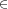## Chapter 14Solutions for third week’s assignments

Also available as PDF.

Note: These are my approaches to these problems. There are many ways to tackle each.

### 14.1 Induction: Sum of first n integers

Prove the formula for the sum of the first n positive integers by mathematical induction. That is, prove 1 + 2 + 3 + \mathrel{⋯} + n = n(n + 1)∕2. The base case here is n = 1. Then show that the nth term transforms into the (n + 1)st term by adding n + 1.

Theorem: Let n be a positive integer. Then the sum of the first n positive integers is

 {\mathop{∑ }}_{i=1}^{n}i = {n(n + 1)\over 2} .

Proof: We proceed by induction. First note that

 {\mathop{∑ }}_{i=1}^{1}i = 1 = {1(1 + 1)\over 2} .

Now assume that

 {\mathop{∑ }}_{i=1}^{n}i = {n(n + 1)\over 2} .

We must show that

 {\mathop{∑ }}_{i=1}^{n+1}i = {(n + 1)(n + 2)\over 2} .

To proceed, we pull the n + 1 term out of the sum to see that

 {\mathop{∑ }}_{i=1}^{n+1}i = n + 1 +{ \mathop{∑ }}_{i=1}^{n}i = (n + 1) + {n(n + 1)\over 2} .

Then we factor out n + 1 and simplify to obtain

\eqalignno{ (n + 1) + {n(n + 1)\over 2} & = (n + 1)\left (1 + {n\over 2} \right ) & & \cr & = (n + 1){2 + n\over 2} & & \cr & = {(n + 1)(n + 2)\over 2} , & & }

proving the result. □

### 14.2 Problem set 2.1 (p83)

#### 14.2.1 Problem 1

Wikipedia can be very useful.

Part a. \{\text{California},\text{Oregon},\text{Idaho},\text{Utah},\text{Arizona}\}. (Note that we could include Nevada; it certainly shares a border with itself.)
Part b. \{\text{Mississippi},\text{Missouri},\text{Maine},\text{Montana},\text{Maryland},\text{Massachusetts},\text{Michigan},\text{Minnesota}\}
Part c. \{\text{Arizona}\}

(Thanks to Chris Fields for pointing out the states I missed.)

#### 14.2.2 Problem 2

Part a. \{\text{a, c, e, h, i, l, m, n, o, s, t, y}\}, possibly including capital “L” if you treat that as a separate letter.
Part b. \{\text{a, e, m, t}\}.

#### 14.2.3 Problem 4

Part a. \{x|xJ,10 < x < 15\}, or perhaps \{10 + i|iJ,0 < i < 5\}.
Part b. \{2i|iJ,3 ≤ i ≤ 8\}.
Part c. \{4 + 4i|iJ,0 ≤ i < 5\}.
Part d. \{{i}^{2} + 2i + 2|iJ,0 ≤ i < 4\} or
\{1 + i|i = \text{ the sum of the first $j$ positive odd integers where $j ≤ 4$}\}

#### 14.2.4 Problem 5

Part a. \{2i|iJ,i > 6\}.
Part b. \{{(2i + 1)}^{2}|iJ,i ≥ 12\}.
Part c. \{3i|i{J}^{+}\}

#### 14.2.5 Problem 6

Part a. No, there are many cities with each name.
Part b. Yes, the cities are specific. There is only one Idaho, Montana, or Texas in the world. There are two Georgias, but only one has a town called Duluth. (The other Georgia does not use the same alphabet, but I am pretty sure no town there transliterates to Duluth.)
Part c. No, there is no universal measure of “smart”.
Part d. Yes, this is specific. However, this likely is empty. How many people have a gpa of exactly 3.5? If you have an odd number of credits, that is not possible. And if you have an even number, you would have to have exactly as many As as Bs.

#### 14.2.6 Problem 27

These are just my rambling responses.

A set of china is a collection of dishware. However, there are many repeated elements that are identical (until chipped), so this is not entirely a mathematical set. If you label each piece, however, then each element has its own identity and you do have a set.

In a family, pretty much everyone has their own identity and is not repeated, even if they are twins. So families could be considered sets. If you rank the family by age or otherwise, the ranked family is ordered and thus not a set.

An aggregate in my mind is a summary and not a listing, but I’m poisoned by programming. So to me, an aggregate is a function you apply to a set to find a value. For example, the mean or the median is an aggregate. But I’m sure there are other uses.

A class in a class room definitely is a set. Each member is unique and identifiable. And by the time there is a final ranking (grade), the class is over. Mathematically, however, class means something beyond set. The collection of all sets is a class and not a set; no set can contain itself as an element.

In my wife’s collection of antique sewing machines, each is identifiable and appears unique. Thus, a set.